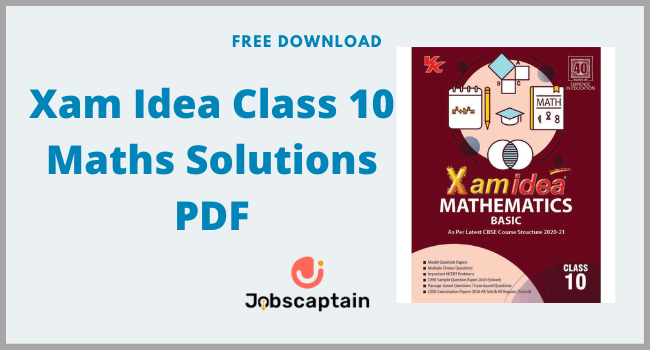By | June 25, 2022Searching for Xam Idea Class 10 Maths Solutions PDF. In this post, we are going to share with you the 10th Class Maths Solution, which XamIdea provides.

Xam Idea Class 10 Maths Solutions have been prepared by expert Maths teachers for the benefit of class 10 students. All the chapters of each book are divided into 2 sections: Part A and Part B. Part-A covers basic concepts, important NCERT textbooks and part B covers periodic tests, Blue Prints, CBSE Examination papers3, and other Question papers.

Also here you can Download Free PDF of Xam Idea Class 10 Book Solutions Below. Let’s read some basic details first:

 Topic Name Xam Idea Mathematics Class 10 Language English Syllabus Central Board of Secondary Education, Delhi Useful for CBSE class 10 and competitive Exams Publisher VK Global publications Pvt. Ltd.
Content Index

## Xam Idea Mathematics Class 10 Syllabus

Here is Some Basic Syllabus Information about Xam Idea Mathematics Class 10:

 No. Subject Marks 1 Number Systems 06 2 Algebra 20 3 Coordinate Geometry 06 4 Geometry 15 5 Trigonometry 12 6 Mensuration 10 7 Statistics & Probability 11 Total Marks 80

## Contents of Xam Idea Mathematics Class 10

Here Some Besic Contents Information about Xam Idea Mathematics Class 10:

### Part: A

• Unit 1: Number Systems
• Real Number
• Unit 2: Algebra
• Polynomials
• Pair of linear equations in two variables
• Arithmetic progressions
• Unit 3: Coordinate Geometry
• Coordinate geometry
• Unit 4: Geometry
• Triangles
• Circles
• Constructions
• Unit 5: Trigonometry
• Introduction to Trigonometry
• Heights and Distance
• Unit 6: Mensuration
• Areas Related to Circles
• Surface Areas and Volumes
• Unit 7: Statistics & Probability
• Statistics
• Probability

### Part: B

• periodic test – 1
• Pen Paper Test
• Multiple Assessment
• periodic test – 2
• Pen Paper Test
• Multiple Assessment
• periodic test – 3
• Pen Paper Test
• Multiple Assessment
• CBSE Sample Question Papers
• Blue Prints
• Model Question papers
• CBSE Examination papers3